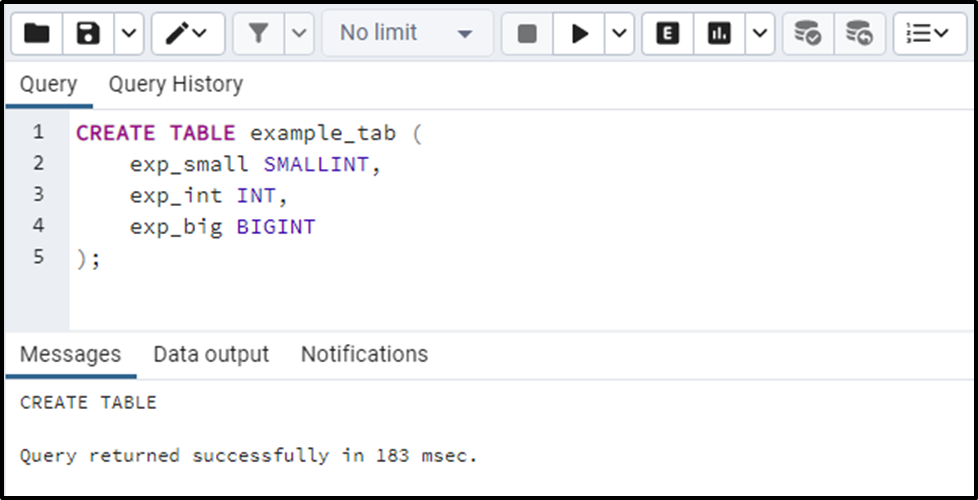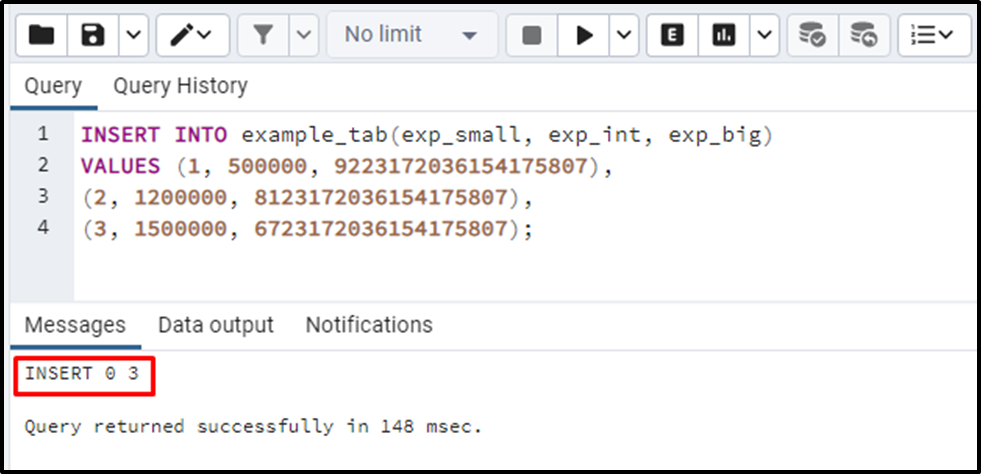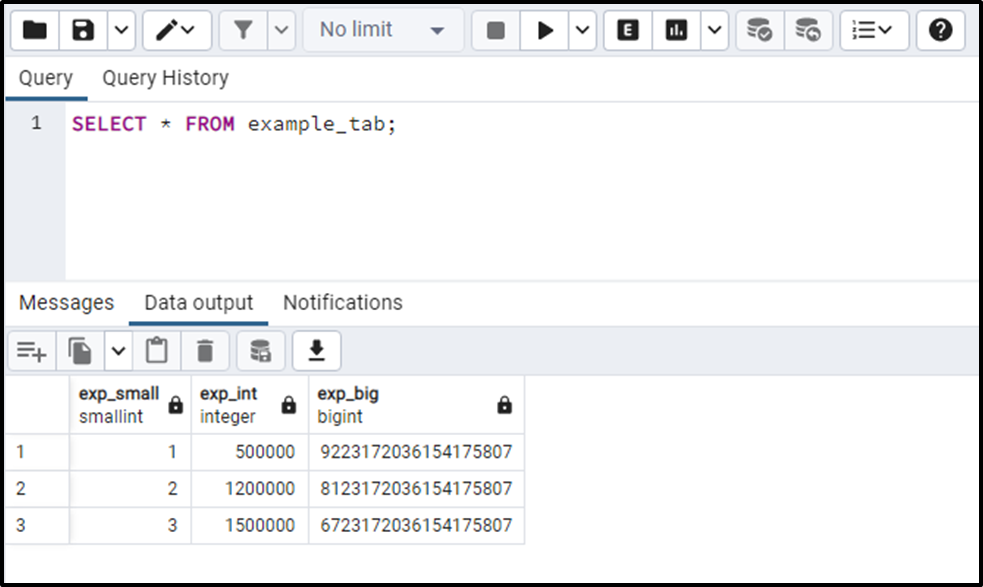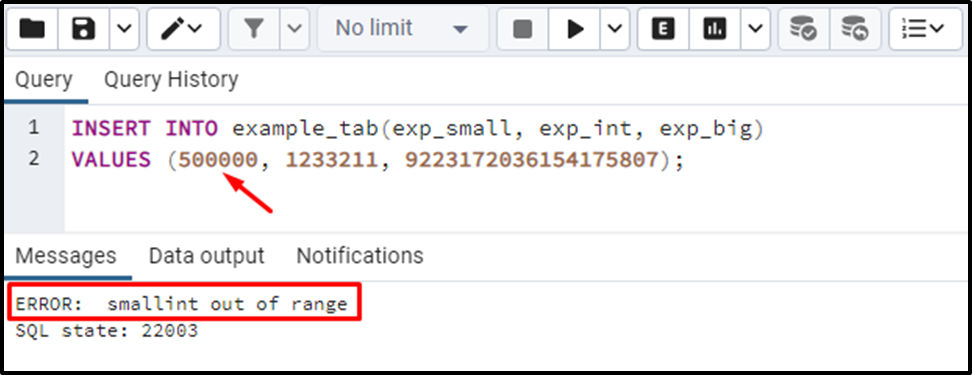##### INTEGER Data Types in PostgreSQL

PostgreSQL provides several integer data types that help us fulfill our needs, such as INTEGER, SMALLINT, and BIGINT. These data types assist us in storing and manipulating whole numbers. Each data type stores a different range of values and has different storage requirements. You can store integer data types in PostgreSQL no matter what your requirements are.

This blog post will explain various integer data types via practical examples. So, let’s start.

How to Use INTEGER Types in Postgres?

This section will explain the three primary data types to store the integer data in Postgres.

SMALLINT Data Type

The below-listed points demonstrate the working of the Postgres SMALLINT data type:

- The storage size of the SMALLINT is two bytes.
- The minimum range of the SMALLINT type is -32,768.
- Its maximum range is +32,767.
- Trying to store an out-of-the-specified range will throw an error.

INT Data Type

The below-listed points present the working of the Postgres INTEGER data type:

- The storage size of the INTEGER is four bytes.
- The minimum range of the INT type is -2,147,483,648.
- Its maximum range is +2,147,483,647.
- Trying to store an out-of-the-specified range will throw an error.

BIGINT Data Type

The following points will explain the working of the Postgres BIGINT data type:

- The storage size of the BIGINT is eight bytes.
- The minimum range of the BIGINT type is -9,223,372,036,854,775,808.
- The maximum range of the BIGINT type is 9,223,372,036,854,775,807.
- Trying to store an out-of-the-specified range will throw an error.

Note: The BIGINT data type consumes too much storage, which decreases the database efficiency. So, use the BIGINT data type when you have a proper reason to use it.

Example: How to Create/Define a Table With INTEGER Data Types in PostgreSQL?

Let’s learn how we can use integer data types in Postgres:

```CREATE TABLE example_tab (
exp_small SMALLINT PRIMARY KEY,
exp_int INT,
exp_big BIGINT,
);```The output snippet indicates that a table has been created with the integer data types. Execute the below query to insert integer data into the “example_tab” table:

```INSERT INTO example_tab(exp_small, exp_int, exp_big)
VALUES (1, 500000, 9223172036154175807),
(2, 1200000, 8123172036154175807),
(3, 1500000, 6723172036154175807);```The output shows that three records have been inserted into the “example_tab” tab. To fetch the “example_tab” data, you must execute the “SELECT *” command:Output authenticates the working of the integer data types.

Example 2: Out-of-Range Error

If we insert an out-of-range value, then we will encounter the following error:

```INSERT INTO example_tab(exp_small, exp_int, exp_big)
VALUES (500000, 1233211, 9223172036154175807);```The maximum range of the SMALLINT type is +32,767; however, we tried to store “500000”, which causes the “smallint out of range” error.

That’s it from this blog.

Conclusion

PostgreSQL provides several integer data types that help us fulfill our needs, such as INTEGER, SMALLINT, and BIGINT. These data types assist us in storing and manipulating whole numbers. Each data type stores a different range of values and has different storage requirements. This post presented a detailed guide on how to use integer data types in Postgres via examples.